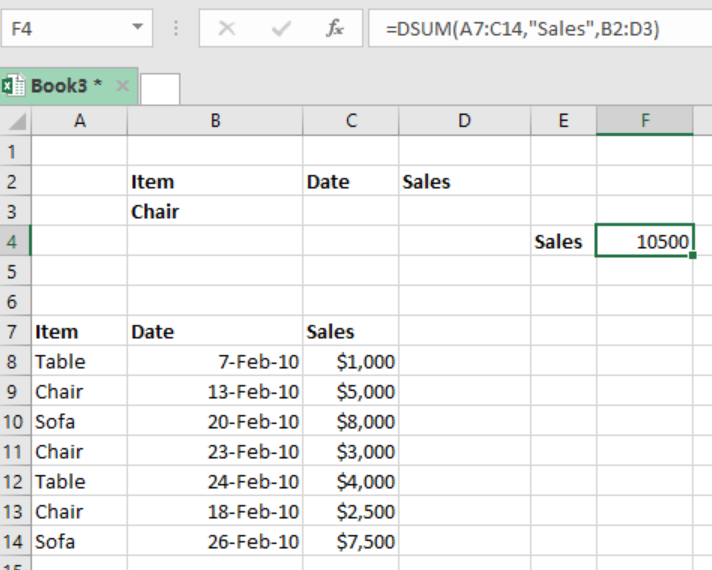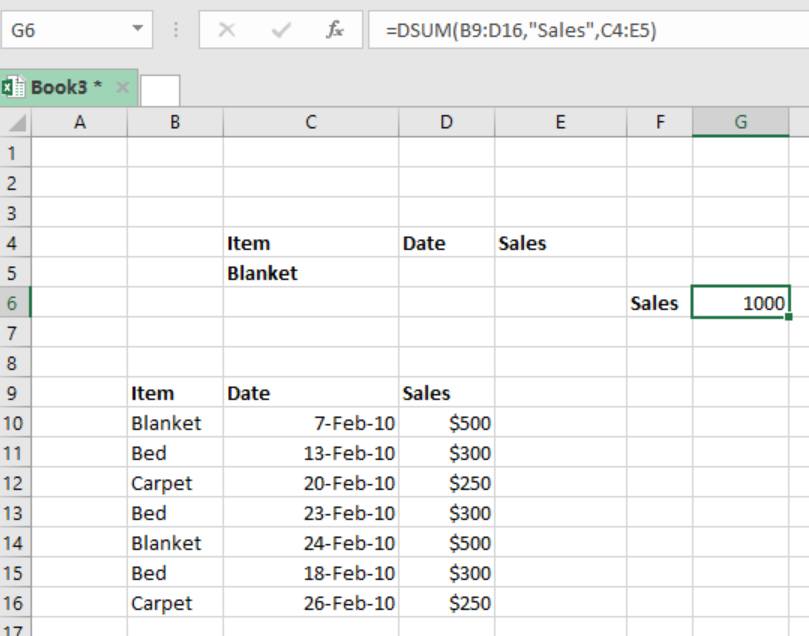Get instant live expert help with Excel or Google Sheets“My Excelchat expert helped me in less than 20 minutes, saving me what would have been 5 hours of work!”

#### Post your problem and you’ll get Expert help in seconds.

Your message must be at least 40 characters
Our professional Expert are available now. Your privacy is guaranteed.

# A Guide on the Excel DSUM Function

If we want to get the sum of given records that match a given specified criteria, we can use the excel DSUM function. In this article, we will provide a clear guide on how use the excel DSUM function.Figure 1: How to use the DSUM function in excel

## General syntax of the formula

`=DSUM(database, field, criteria)`

Where;

Database- refers to the data range including the headers

Field- refers to the field name or the index to count

Criteria– criteria to use in the range including the headers

## Understanding the formula

• We use this function to calculate the sum of values that are in a set of records that match our given criteria.
• This formula sums the values that have been extracted from a certain field in the supplied database.
• When working with this function you can use a variety of expressions, including wildcards.

## Using the multi-row criteria

It is important to know that the DSUM function can include more than one row below the headers. In this case, each row needs to be joined by the OR logic. Also, the expressions in the given criteria will be joined by the AND logic.

### ExampleFigure 2: Example of how the DSUM function is used to find sum for different dates

In this example, we want to find the sum of blanket sales in two different dates. To do this, we proceed as follows;

Step 1: Tabulate the table with the data

Step 2: Indicate where you want to have your answer, in our case, we want the answer in cell G6.

Step 3: in cell G6, input the formula `=DSUM(B9:D16,"Sales",C4:E5)`

Step 4: press enter to get the sum of the price of blanket in two different dates.

## Instant Connection to an Expert through our Excelchat Service

Most of the time, the problem you will need to solve will be more complex than a simple application of a formula or function. If you want to save hours of research and frustration, try our live Excelchat service! Our Excel Experts are available 24/7 to answer any Excel question you may have. We guarantee a connection within 30 seconds and a customized solution within 20 minutes.

### Did this post not answer your question? Get a solution from connecting with the expert.Another blog reader asked this question today on Excelchat:
Solution examplesHi, I am trying to find an average of one column 'C', where another column = a particular value, or a third column = another particular value.
Solved by T. C. in 19 minsI need a formula where s36 = 1 if a10=s7 and c10=any value?
Solved by G. J. in 13 minsHello, I need help. (Excel) Assume that columns A, C and E in a worksheet contain the names of three adults who are interested in joining the (AARP), 50 years of age and over improve the quality of their lives. Columns B, D and F contain their respective ages in whole years in the adjacent cells. Which of the following formulas should be placed in cell G1 to verify that anyof these individuals are eligible to become full members, if they are at least 50 years old? =AND(B1>49, D1>49, F1>49) =OR(B1>=50, AND(D1>=50, F1>=50)) =OR(B1>=50, D1>=50, F1>=50) =AND(B1>=50, D1>=50, F1>=50
Solved by F. Q. in 20 minsI need a long If and AND statement. If a1 is between 17-21 AND a2 is greater than 42 and a3 is greater 53 and a4 is less than the time of 15:54 then they pass, if not they fail
Solved by E. S. in 54 minsWould like to know how use the filter in excel with AND instead of OR So filtering three columns with a 0 and 1 and asking that all three show up if at least one has a number 1
Solved by B. E. in 20 mins## Subscribe to Excelchat.coAnother blog reader asked this question today on Excelchat: